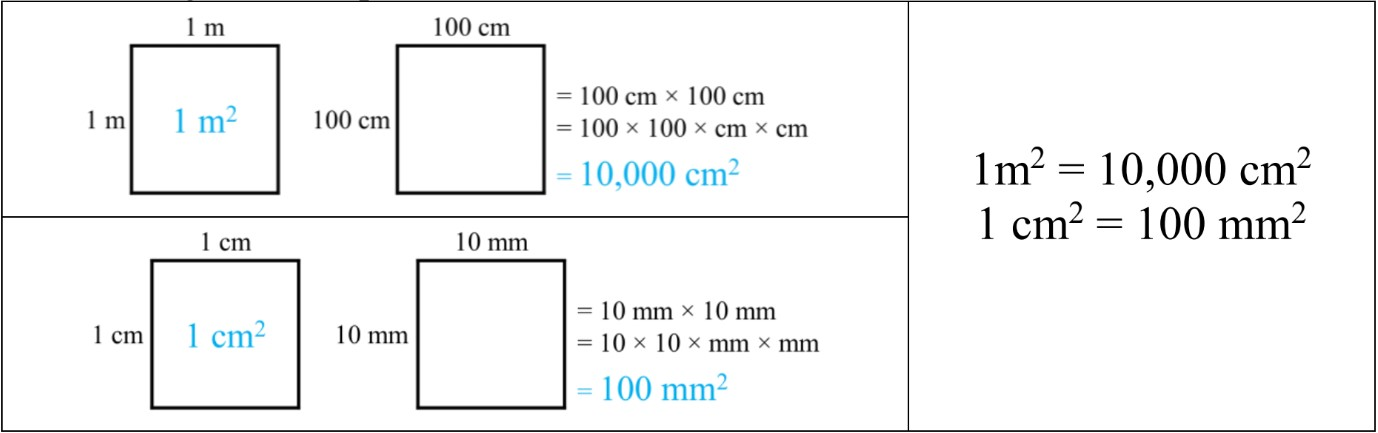# Square measure#### All in One Place

Everything you need for JC, LC, and college level maths and science classes.#### Learn with Ease

We’ve mastered the national curriculum so that you can revise with confidence.#### Instant Help

24/7 access to the best tips, walkthroughs, and practice exercises available.

0/4
##### Intros
###### Lessons
1. Introduction to Square Measure:
2. What is square measure?
3. Different types of square units
4. Review: converting between single units
5. Converting between square units
0/10
##### Examples
###### Lessons
1. Comparing different square measures: mm2, cm2, m2
Fill in the blanks for the proper unit for each object: mm2, cm2, or m2.
1. The classroom wall measures 15 ___.
2. A sheet of lined paper measures 616 ___.
3. One face on a die measures 144 ___.
2. Calculate the area of a figure made of unit squares
Calculate the area of each figure. Give the answer with the proper units for each unit square.
1.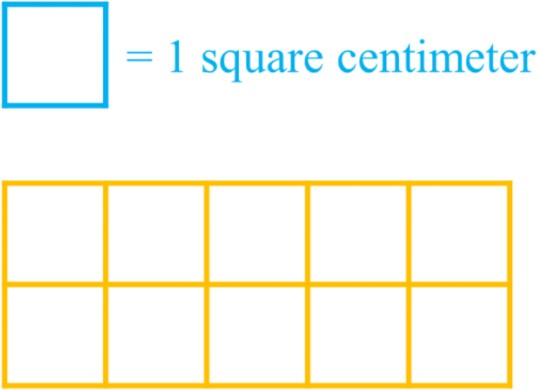2.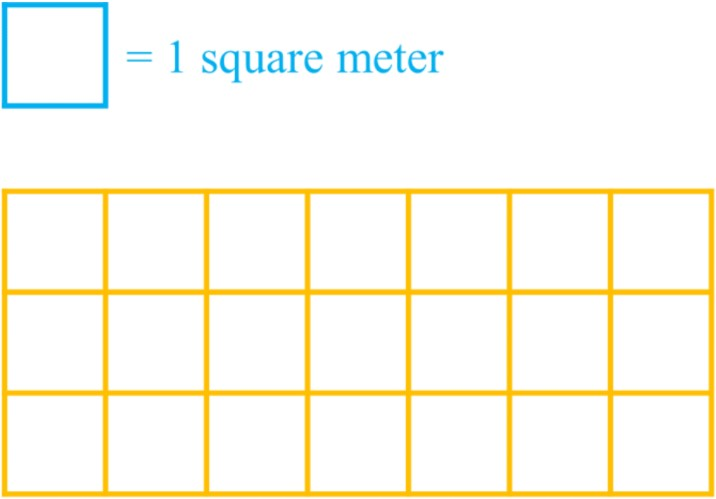3.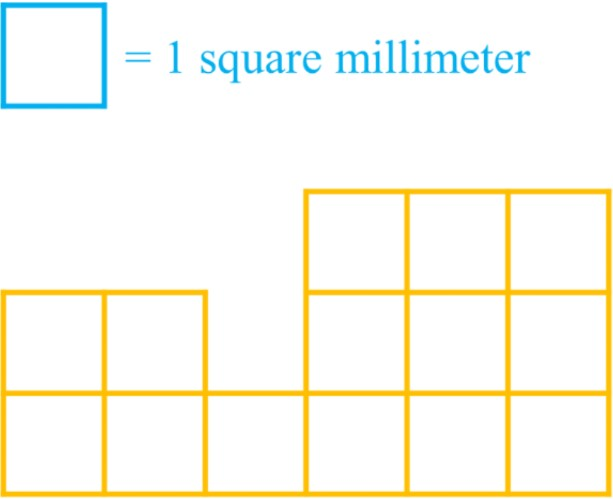3. Area of figures drawn on grid paper
Using the centimeter grid paper, draw a rectangle figure with an area of:
1. 4 cm2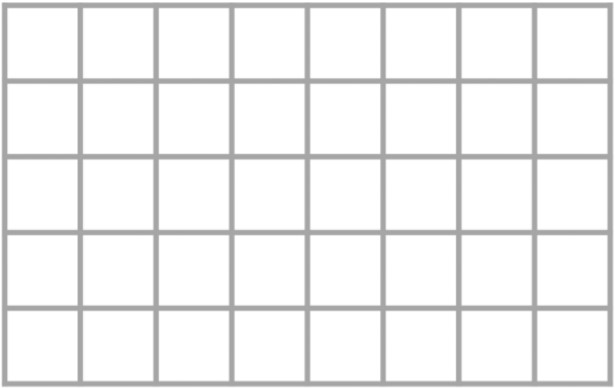2. 24 cm2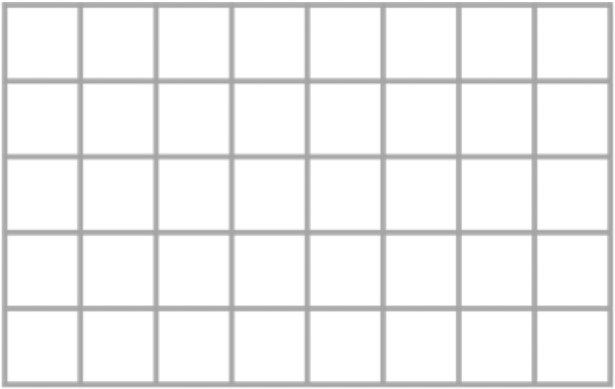4. Convert between square units using conversion facts
Convert the square units by using the conversion fact.
1. If there are 10,000cm2 in 1m2, how many cm2 are there in 6m2?
10,000cm2 = 1m2
_____ cm2 = 6m2
2. If there are 100mm2 in 1cm2, how many cm2 are there in 800mm2?
100mm2 = 1cm2
800mm2 = __ cm2
###### Free to Join!
StudyPug is a learning help platform covering math and science from grade 4 all the way to second year university. Our video tutorials, unlimited practice problems, and step-by-step explanations provide you or your child with all the help you need to master concepts. On top of that, it's fun - with achievements, customizable avatars, and awards to keep you motivated.
• #### Easily See Your ProgressWe track the progress you've made on a topic so you know what you've done. From the course view you can easily see what topics have what and the progress you've made on them. Fill the rings to completely master that section or mouse over the icon to see more details.
• #### Make Use of Our Learning Aids###### Practice Accuracy

Get quick access to the topic you're currently learning.

See how well your practice sessions are going over time.

Stay on track with our daily recommendations.

• #### Earn Achievements as You LearnMake the most of your time as you use StudyPug to help you achieve your goals. Earn fun little badges the more you watch, practice, and use our service.
• #### Create and Customize Your AvatarPlay with our fun little avatar builder to create and customize your own avatar on StudyPug. Choose your face, eye colour, hair colour and style, and background. Unlock more options the more you use StudyPug.
###### Topic Notes

In this lesson, we will learn:

• Square measure refers to the squared units that are used to measure area
• How to count the square measure of areas shown by unit square figures
• Comparing between metric units and squared metric units

Notes:

• Square measure refers to the units we use to measure area
• A unit square is a square with a measure of 1 unit on each side
• Units can be in metric units (i.e. m2, cm2, mm2) or other customary units (i.e. ft2, in2, yd2)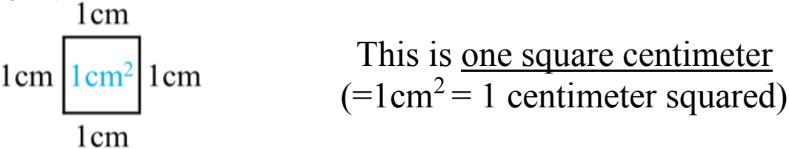• Different dimensions (1-dimensional or 2-dimensional) correspond to geometric properties of shapes (perimeter or area) as well as their units: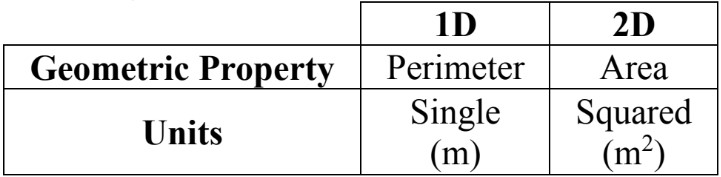• The square measure of area can be thought of as the number of unit squares that cover the 2D shape. You can count each unit square one by one to find the area.
• If you have a rectangular figure of unit squares, think of it as an array: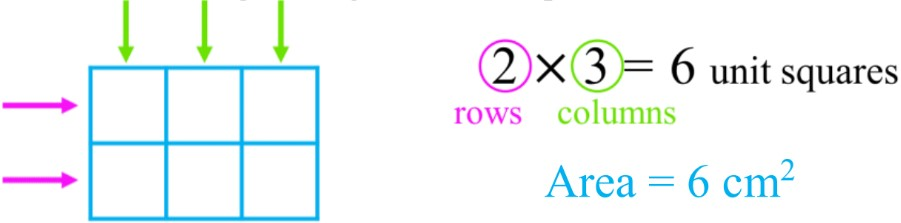• Converting between metric units: meters (m), centimeters (cm), millimeters (mm)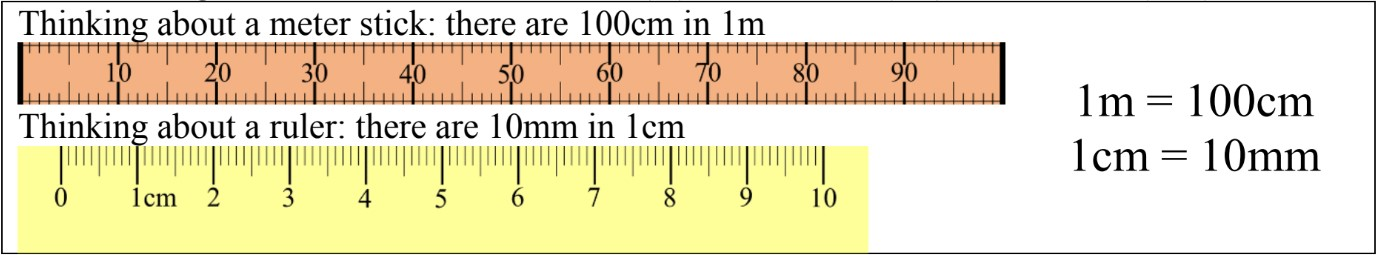• Converting between squared metric units: m2, cm2, mm2